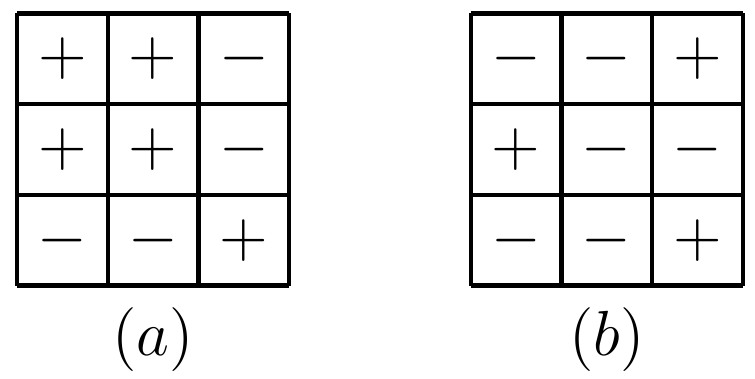#### Russia/USSR

###### back to index

Find any positive integer solution to $x^2 - 51y^2 = 1$.

Solve in integers $\frac{x+y}{x^2-xy+y^2}=\frac{3}{7}$

For any arithmetic sequence whose terms are all positive integers, show that if one term is a perfect square, this sequence must have infinite number of terms which are perfect squares.

Find all positive integer $n$ such that $(3^{2n+1} -2^{2n+1}- 6^n)$ is a composite number.

Find $n$ different positive integers such that any two of them are relatively prime, but the sum of any $k$ ($k < n$) of them is a composite number.

Solve this equation in positive integers $$x^3 - y^3 = xy + 61$$

Show that the following inequality holds for any positive integer $n$: $$(2n+1)^n \ge (2n)^n + (2n-1)^n$$

Let $a, b, c, m, n, p, k$ be positive real numbers that satisfy $a+m = b+n = c+p=k$. Show that $an+bp+cm < k^2$.

Let $a_1=a_2=1$ and $a_{n}=(a_{n-1}^2+2)/a_{n-2}$ for $n=3, 4, \cdots$. Show that $a_n$ is an integer for $n=3, 4, \cdots$.

Show that if a polynomial $P(x)$ satisfies $P(2x^2-1)=(P(x))^2/2$, then it must be a constant.

Is it possible to construct 12 geometric sequences to contain all the prime between 1 and 100?

Is function $f(x)=\lg(x+\sqrt{x^2+1})$ an odd or even function?

Let $a$ and $b$ be two positive real numbers not exceeding $1$. Prove $$\frac{1}{\sqrt{a^2 + 1}}+\frac{1}{\sqrt{b^2 +1}}\le\frac{2}{\sqrt{1+ab}}$$

Given two grids shown below, is it possible to transform (a) to (b) after a series of operations? In each operation, one can change all the signs in either one entire row or one entire column.